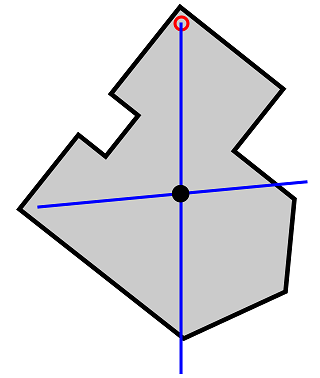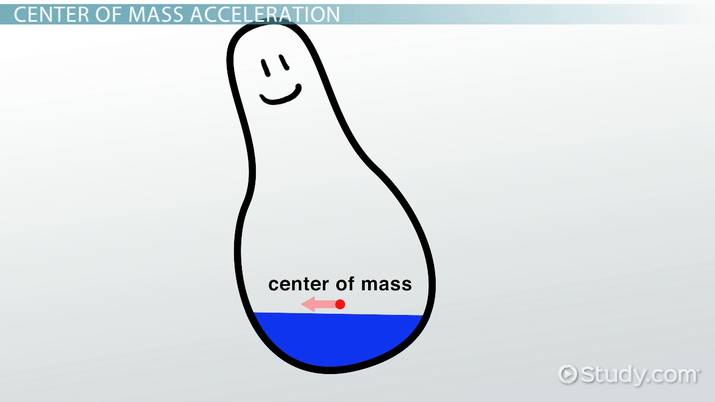# Center of Mass

Chelsea Coons, Matthew Bergstresser
• Author
Chelsea Coons

Chelsea has taught middle school math and elementary school (all subjects) for 7 years. She has a Master's Degree in Educational Leadership and Administration and a Bachelor's Degree in Elementary Education with a concentration in Mathematics from Ball State University..

• Instructor
Matthew Bergstresser

Matthew has a Master of Arts degree in Physics Education. He has taught high school chemistry and physics for 14 years.

Understand how to calculate the velocity of the center of mass using an equation. Learn how the acceleration of the center of mass relates to the velocity. Updated: 02/28/2022

Show

## Center of Mass Formula

The center of mass is the average position of all the particles within an object or system of objects. For simple objects with constant densities, finding the center of mass can be intuitive. A square's center of mass is in the geometric center of the square, also known as the centroid, because that is the average position of all the square's mass. However, sometimes more complex shapes or multiple objects in a system make it difficult to know where the center of mass is.The center of mass formula for a system of objects (in this case, three objects) can be found using: {eq}X = \frac{m1*x1*m2*x2*m3*x3}{m1+m2+m3} {/eq}

• X = the position of the center of mass
• m = the mass of an object (kg)
• x = the position of an object

Note: the position unit can vary as long as it remains consistent throughout the work.An error occurred trying to load this video.

Try refreshing the page, or contact customer support.

Coming up next: Uniform Circular Motion: Definition & Mathematics

### You're on a roll. Keep up the good work!

Replay
Your next lesson will play in 10 seconds
• 0:03 Center of Mass Velocity
• 0:56 Center of Mass Acceleration
• 1:48 Three Masses in Space
• 3:45 An Example
• 5:37 Lesson Summary
Save Save

Want to watch this again later?

Timeline
Autoplay
Autoplay
Speed Speed

## Velocity of Center of Mass

Velocity is the change in an object's position over time. Since the center of mass moves along with an object or system of objects in motion, the overall movement can be described in terms of the velocity of center of mass.

## Velocity of Center of Mass Equation

Staying with the three-object-system that was used in the previous formula, the velocity of center of mass equation is the sum of the momentum of the three objects in the system, divided by the total mass:

{eq}V_{cm} = \frac{(m_1v_1) + (m_2v_2) + (m_3v_3)}{m_1+m_2+m_3} {/eq}

• V = velocity of the center of mass (m/s)
• m = the mass of an object (kg)
• v = velocity of an object (m/s)

Let's say there are three objects. One with a mass of 2 kg, one with a mass of 5 kg, and one with a mass of 8 kg. The first object has a velocity of 1 m/s, the second has a velocity of 4 m/s, and the last has a velocity of 2 m/s. The velocity of the center of mass can be found using the formula above.

{eq}V_{cm} = \frac{(2 kg*1 m/s) + (5 kg*4 m/s) + (8 kg*2 m/s)}{2 kg+5 kg+8 kg} {/eq}

Simplified, this equals:

{eq}V_{cm} = \frac{38 kg*m/s}{15 m/s} {/eq}

Which equals approximately 2.5 m/s for the velocity for the center of mass.

## Acceleration of Center of Mass

Acceleration occurs when velocity changes in relation to time. Acceleration of an object can be found using the formula:

F = m * a

F = force (N)

m = mass (kg)

a = m/s^2

The acceleration of center of mass can be found using the formula:

{eq}a_{cm} = \frac{m_1a_1 + m_2a_2 + ... + m_na_n}{m_1 + m_2 + ... m_n} = \frac{m_1a_1 + m_2a_2 + m_na_n}{M} {/eq}

• The acceleration of the center of mass is {eq}a_{cm} {/eq}
• The acceleration of particle 1, etc. is {eq}a_1 {/eq}
• The mass of particle 1, etc. is {eq}m_1 {/eq}
• The total mass is M

Multiplying both sides by M gives:

{eq}Ma_{cm} = m_1a_1 + m_2a_2 + ... + m_na_n {/eq}

• {eq}m_1a_1 {/eq} is the net force of particle 1
• {eq}m_2a_2 {/eq} is the net force of particle 2
• {eq}m_na_n {/eq} is the net force of n particles

Example:

In this example there are three objects. The mass of object 1 is 5 kg. The mass of object 2 is 2 kg. The mass of object 3 is 8 kg. The acceleration for object 1 is 2 m/s. The acceleration for object 2 is 1 m/s The acceleration for object 3 is 5 m/s.

Start with the formula {eq}a_{cm} = \frac{m_1a_1 + m_2a_2 + ... + m_na_n}{m_1 + m_2 + ... m_n} = \frac{m_1a_1 + m_2a_2 + m_na_n}{M} {/eq}

Plug in the known values {eq}a_{cm} = \frac{5*2 + 2*1 + 3*5}{5 + 2 + 3} {/eq}

Simplify {eq}a_{cm} = \frac{10 + 2 + 15}{10} {/eq}

{eq}a_{cm} = \frac{27}{10} {/eq} or 2.7 m/s

To unlock this lesson you must be a Study.com Member.

#### What is center of mass simple?

The center of mass is simple the geometric center of the shape. For more complex shapes, the center of mass formula must be used.

#### What is the formula for center of mass?

The formula for the center of mass is the sum of the product of the mass and position of each particle in the system. Take that and divide it by the sum of the mass of each particle.

#### How do you find the center of mass?

To find the center of mass of a system of particles, you must know the mass and position of each particle. Add up the sum of the product of the mass and position of each particle and divide it by the sum of the mass of each particle.

#### Is the velocity of the center of mass constant?

Yes, the velocity of the center of mass is constant. The exception would be if it is acted on by an outside force.

#### What is the center of mass in physics?

The center of mass for a simple object like a square, is its geometric center (centroid). To find the center of a more complex object, the center of mass formula is needed. The formula for a system of particles is the sum of the product of the mass and position of each particle, divided by the total mass.

#### Is there a relationship between mass and velocity?

The velocity of the center of mass for a group of objects is the sum of the product of each object's mass and velocity, divided by the total mass. The momentum of an object is the product of its mass and velocity.

### Register to view this lesson

Are you a student or a teacher?

Back

### Resources created by teachers for teachers

Over 30,000 video lessons & teaching resources‐all in one place.Video lessonsQuizzes & WorksheetsClassroom IntegrationLesson Plans

I would definitely recommend Study.com to my colleagues. It’s like a teacher waved a magic wand and did the work for me. I feel like it’s a lifeline.

Jennifer B.
TeacherCreate an account to start this course today
Used by over 30 million students worldwide